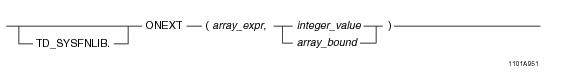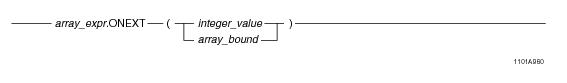## SQL Functions, Operators, Expressions, and Predicates

Product
Release Number
15.00
Language
English (United States)
Last Update
2018-09-24
dita:id
B035-1145
lifecycle
previous
Product Category

## ONEXT

### Purpose

Returns the subscript of the element subsequent to the element specified for array_expr, if that element is currently populated.

### Syntax

System function syntax:Method-style syntax:where:

 Syntax element… Specifies… TD_SYSFNLIB the name of the database where the method is located. array_expr an ARRAY expression, which is one of the following: A reference to an ARRAY column An ARRAY constructor expression. See “ARRAY Constructor Expression” on page 195. A UDF expression A UDM expression integer_value an unsigned INTEGER value. array_bound an array instance of the predefined array type ArrayVec with a comma-separated list of integer values to define the bounds. For details, see “ARRAY Scope Reference” on page 198.

### ANSI Compliance

This is a Teradata extension to the ANSI SQL:2011 standard.

### Usage Notes

ONEXT takes an array expression as an argument and returns the subscript of the element subsequent to the element specified by integer_value or array_bound, if that element is currently populated. If you specify an element subscript that is greater than or equal to the value of OLAST for the array, a NULL is returned. Also, if integer_value or array_bound is NULL, an error is returned.

If array_expr is a one-dimensional ARRAY type, ONEXT returns an unsigned INTEGER value. If array_expr is a multidimensional ARRAY type, ONEXT returns a new instance of the predefined ARRAY type ArrayVec, containing the subscript information. If the array is empty (all elements of the array are in an uninitialized state), then ONEXT returns NULL.

If array_expr is NULL, an error is returned.

The ONEXT method with one INTEGER argument is compatible with the Oracle NEXT method for one-dimensional ARRAY types.

### Result Type

ONEXT returns an unsigned INTEGER value or a new instance of the predefined ARRAY type ArrayVec.

### Example

Consider the following one-dimensional ARRAY data type and table.

`CREATE TYPE phonenumbers AS VARRAY(20) OF CHAR(10);`
` `
`CREATE TABLE employee_info (eno INTEGER, phonelist phonenumbers);`

The table is populated with the following values:

`/* The first 2 elements are populated; the rest are uninitialized. */`
`INSERT INTO employee_info VALUES (1, `
`   phonenumbers('1112223333', '6195551234'));`
` `
`/* Empty ARRAY instance */`
`INSERT INTO employee_info VALUES (2, `
`   phonenumbers());`
` `
`/* Update empty ARRAY instance such that element 3 is set to a value;`
`   Then elements 1 and 2 are set to NULL, the rest are uninitialized */`
` `
`UPDATE employee_info`
`SET phonelist = '8584850000'`
`WHERE id = 2;`

The following query returns the subscript of the element subsequent to element 2 in the phonelist array.

`SELECT eno, phonelist.ONEXT(2)`
`FROM employee_info;`

The following is the result of the query.

`ENO         phonelist.ONEXT(2)`
`---         ------------------`
` 1           ?         (the element following element 2 is unpopulated) `
` 2           3`

### Example

Consider the following 2-D ARRAY data type and table.

`CREATE TYPE shot_ary AS VARRAY(1:50)(1:50) OF INTEGER;`
` `
`CREATE TABLE seismic_table (`
`   id INTEGER,`
`   shots shot_ary);`

The table is populated with the following values:

`/* The first 2 elements are populated; the rest are uninitialized. */`
`INSERT INTO seismic_table VALUES (1, shot_ary(11, 12));`
` `
`/* Empty ARRAY instance */`
`INSERT INTO seismic_table VALUES (2, shot_ary());`
` `
`/* Update empty ARRAY instance such that element  is set to a value; Then elements  and  are set to NULL, the rest are uninitialized */`
` `
`UPDATE seismic_table`
`SET shots = 1133`
`WHERE id = 2;`

The following query returns the subscript of the element subsequent to element  in the shots array.

`SELECT id, shots.ONEXT(NEW arrayVec(1, 2)) `
`FROM seismic_table;`

The following is the result of the query.

`ID          shots.ONEXT(NEW arrayVec(1, 2))`
`--          -------------------------------`
` 1           ?     (The element following element  is unpopulated)`
` 2           NEW arrayVec(1,3)`

The following is the same query using function-style syntax.

`SELECT id, ONEXT(shots, NEW arrayVec(1, 2)) `
`FROM seismic_table;`